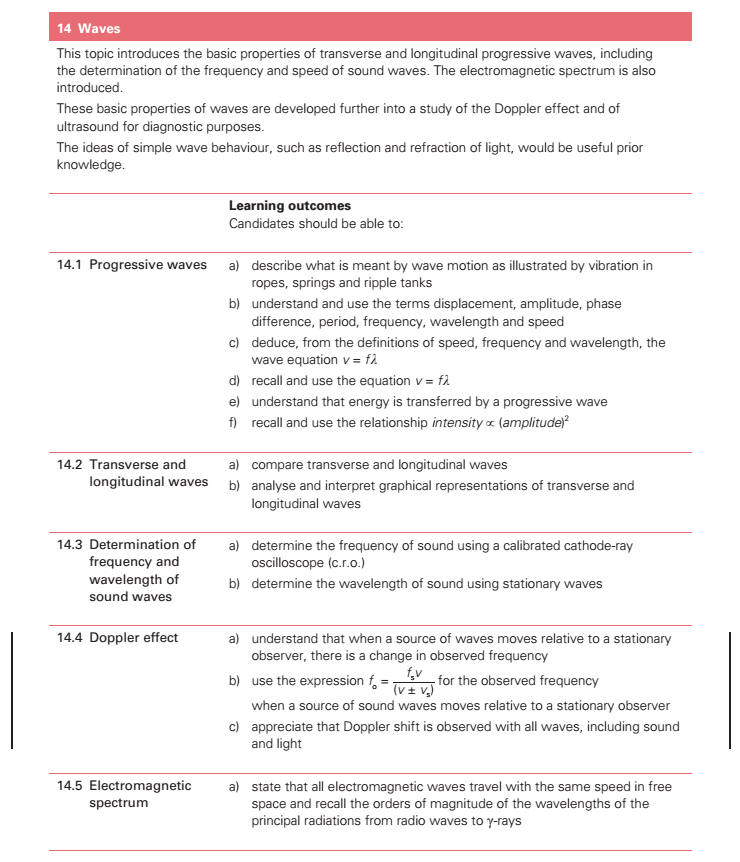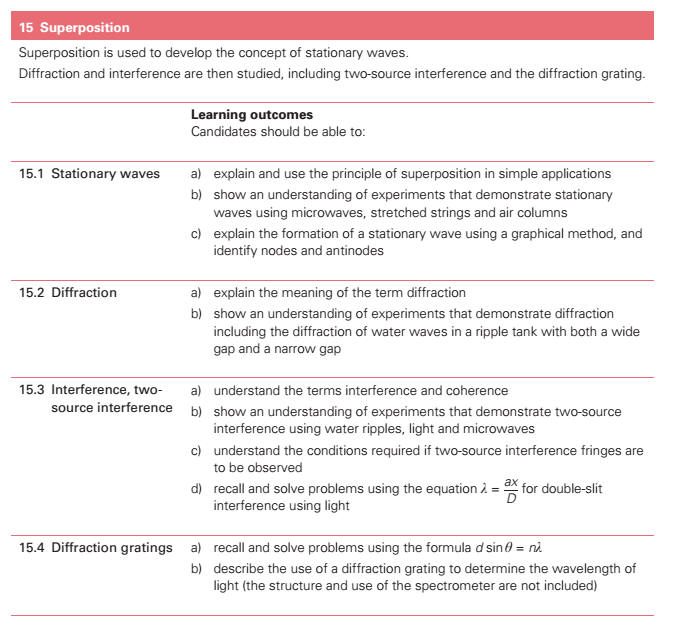14. Waves (a) describe what is meant by wave motion as illustrated by vibration in ropes, springs and ripple tanks. (b) show an understanding and use the terms displacement[], amplitude, phase difference, period[], frequency, wavelength[] and speed. (c) deduce, from the definitions of speed, frequency and wavelength, the equation ν = fλ. (d) recall and use the equation ν = fλ. (e) show an understanding that energy is transferred due to a progressive wave. (f) recall and use the relationship, ∝ (amplitude)2. (g) compare transverse and longitudinal waves. * (h) analyse and interpret graphical representations of transverse and longitudinal waves. (i) show an understanding that polarisation[] is a phenomenon associated with transverse waves. * (j) determine the frequency of sound using a calibrated c.r.o. * (k) determine the wavelength[] of sound using stationary waves. * (l) state that all electromagnetic waves travel with the same speed in free space and recall the orders of magnitude[] of the wavelengths of the principal radiations from radio waves to γ-rays. 15. Superposition[] * (a) explain and use the principle of superposition in simple applications. * (b) show an understanding of experiments which demonstrate stationary waves[] using microwaves, stretched strings and air columns. * (c) explain the formation of a stationary wave using a graphical method, and identify nodes and antinodes. (d) explain the meaning of the term diffraction. (e) show an understanding of experiments which demonstrate diffraction including the diffraction[] of water waves in a ripple tank with both a wide gap and a narrow gap. (f) show an understanding of the terms interference and coherence. (g) show an understanding of experiments which demonstrate two-source interference using water, light and microwaves. (h) show an understanding of the conditions required if two-source interference fringes are to be observed. (i) recall and solve problems using the equation λ = ax / D for double-slit interference using light. (j) recall and solve problems using the formula d sinθ = nλ and describe the use of a diffraction[] grating to determine the wavelength